American Journal of Civil Engineering
Volume 5, Issue 1, January 2017, Pages: 50-59

Modified Three-Parameter Power Model to Predict Moment-Rotation Curve of Top- and Seat-Angle Connection

Ali Ahmed1, *, Norimitsu Kishi2

2Kushiro National College of Technology, Otanoshike, Kushiro, Japan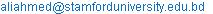(A. Ahmed)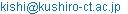(N. Kishi)

*Corresponding author

Ali Ahmed, Norimitsu Kishi. Modified Three-Parameter Power Model to Predict Moment-Rotation Curve of Top- and Seat-Angle Connection. American Journal of Civil Engineering.Vol. 5, No. 1, 2017, pp. 50-59. doi: 10.11648/j.ajce.20170501.17

Received: December 4, 2016; Accepted: December 15, 2016; Published: January 18, 2017

Abstract: This study conducted on improving three-parameter power model  to estimate ultimate moment of connections based on the failure mechanisms developed by more practical means and finally, to predict moment-rotation relations of top- and seat-angle connections for intrinsic replacement of experimental curves and/or finite element (FE) analysis results. Bolt stiffness, shear and bending deformation of tension angle and prying force acted on tension angle are considered to determine the ultimate moment of connections implementing two possible failure mechanisms. These failure mechanisms are developed based on the concept of T-stub model  and adjusted the position of plastic hinges applying advanced FE analysis method [3-6]. Then, moment-rotation (M-qr) characteristics of top- and seat-angle connections are constructed applying proposed modified three-parameter power model. Applicability of the proposed formulation is examined by comparing M-qr curves and ultimate moment capacities with those of Kishi-Chen power model, FE analyses [3, 4] and experiments [7, 8]. The comparison implies that proposed formulation and Kishi-Chen’s method both achieved closer approximation for maximum of cases and better accuracy for the modified cases to drive M-qr curves of the connections; but ultimate moments of the connections defined by the proposed method is more realistic than that predicted by Kishi-Chen power model.

Keywords: Moment-Rotation Relation, Prying Action, Ultimate Moment, Initial Stiffness, Failure Mechanism, Top- and Seat-Angle Connection

1. Introduction

In practice, AISC-LRFD specifications recommend top- and seat-angle connections to the Partially Restrained construction and consider only for transferring beam-shear force. But experimental evidences and advanced FE analyses on the connections expose that besides developing beam-shear force, this type of connections transfer fairly significant beam-end-moment to the column [9,10]. This moment trans-fers to the column through the tension angle and makes an increase in tensile force known as prying force. Prying creates additional force in the bolts due to the deformation of tension angle’s vertical leg. A few researchers have paid serious attention to this additional force in their mathematical representation of connection behavior. Recently, the T-stub modeling concept has been used to represent mechanical behavior of extended end plate connections and modified to use for angle type of connections [11,12,2]. However, the T-stub model is somehow different in respect to representing the actual deformation pattern of true angle type of connections stating from plastic yielding to the failure , and may loose its efficiency in estimation of connection strength.

From that point of view, this study considers improving the moment-rotation model of Kishi and Chen  by incorporating bolt stiffness (but bolt has sufficient strength not to fail before angle failure) and tension angle’s prying action on bolt deformation. In addition, the Kishi-Chen power model has a simple form of representation, is easy to implement in second order frame analysis and is best representing its physical meaning, which motivated studying the model further. Kishi and Chen considered bending and shear deformations of the angle, but disregarded deformation of bolts and prying action on tension angle for deriving the ultimate moment capacity of angle type of connections in their power model. To establish a rational prediction model for representing the M-qr curves of the connections, this effect on connection behavior needs to be considered. The formulation determining the ultimate connection moment Mu is proposed assuming two types of simple mechanisms of connection failure using the T-stub concept of "Eurocode 3" . The locations of plastic hinges in the failure mechanism assumed by the power model  are revised based on the deformation patterns of the connections reproduced by FE analyses [3,4,6] and experiments [5,7,8]. In this formulation the effects of prying action, bolt stiffness, and bending and shear deformations of the tension angle are considered. Replacing ultimate moment of the connection Mu predicted by the proposed formulation, initial connection stiffness Rki estimated by Kishi-Chen’s formulation (Eq. 3) and shape parameter n obtained by a least-mean square fit technique  into the three-parameter power model, M-qr relations of top- and seat-angle connections are reproduced. Performance of the proposed prediction model is assessed by comparing the values of Mu and M-qr curves predicted by the proposed formulation with FE analysis , Kishi-Chen power model  and experimental results [7,8,15]. It is observed that the proposed model and Kishi-Chen power model can generate acceptable M-qr curves for all of the connections except largely deformable connections. But, the proposed method shows better performances in obtaining the ultimate moment of the connections than those estimated by Kishi-Chen power model.

2. FE Analysis of Connections

Advanced finite element analyses of top- and seat-angle connections were performed by the author and their geometrical and mechanical properties and analysis results were discussed elaborately in the literatures [3,4,15,16]. In those articles with the other’s similar investigation [5,6,10], it was observed that plastic yielding of the connections initiated at the toe of the tension angle’s fillet and then followed by yielding in the area under the tension bolt head for thinner angles (e.g., for the cases of ⅜" angle thickness), and in the areas of tension bolthole for the cases of thicker angles. And, it was noted that as the tension angle thickness increases, the zone of plastic yielding moves toward the centreline of the bolthole of the vertical leg. Simultaneously, FE analysis results showed that the stresses higher than the yield point were generated in the bolt shank near the bolt head for most of the connections, which indicates that the nonlinear behavior of the connection differs from some assumptions of power model. Due to that observation, the place of formation of the upper plastic hinge in the tension angle’s vertical leg has been revised and an additional plastic hinge is supplied in the tension bolts according to the results of FE analyses for the proposed prediction model in determining the ultimate connection moment. FE analysis results [3,4,16] also demonstrated that the distance of the prying force acting point from the centerline of bolthole at the ultimate level of loading mostly depended on the thickness of the tension angle and gage distance from angle heel to the centerline of bolthole. Therefore, it was found that the distance b of the prying force acting point from the centerline of bolthole is a function of the angle thickness of tension angle, tt in combination with gage distance from angle heel to the centerline of bolt hole, gt' and expressed as  if (2.575tt – 0.05gt') < a then

b = 2.575tt – 0.05gt' else b = a         (1)

where a is the distance from the centerline of bolt hole to the top edge of tension angle’s leg adjacent to column flange.

In addition, it was defined from FE analysis results that prying force should be considered in the calculation of design resistance of tension fasteners and the ultimate moment of the connection and can be as large as 2.4 times the shear force (i.e., beam flange force).

3. Modified Three-Parameter Power Model

As top- and seat-angle connections are capable of transferring considerable amount of beam end moment, they are treated as semi-rigid connection in the research community and design offices. Since the inception of analysis and design of steel frame structures incorporating semi-rigid connections, several mathematical representations of moment-rotation behavior of semi-rigid connections have been proposed. Among them, linear models were the most straightforward [17-19], but they have not been much improvedover the conventional connection models for limiting with two extremes of rigid and pinned connection. Thus, the linear models were soon surpassed by the introduction of bilinear/piece-wise linear models [20-23]. Frye and Morris proposed the polynomial model formulated using curve-fitting constants . However, the polynomial model has been received considerable progress against the drawbacks of linear/bilinear/piecewise linear models; producing negative stiffness makes the model less attractive for the implementation in a computer based analysis program. Lui and Chen  proposed a multi-parameter exponential model, which was further refined by Kishi and Chen . Kishi and Chen later made an improvement by introducing a semi-analytical model . The model used a power function similar to those of Richard and Abbott  and Colson and Louveau  and employed three-parameters, namely, initial connection stiffness Rki, ultimate moment capacity Mu, and a shape parameter n. The three-parameter power model is mathematically represented by the following equation:(2)

where M and qr are moment and relative rotation, respectively; qo is a reference plastic rotation; qo = Mu/Rki, in which Rki is initial connection stiffness given by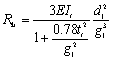(3)

where EIt is the bending stiffness of the tension angle’s vertical leg; g1 = gt' – 0.5wb – 0.5tt; gt' is the gage distance from the heel to the bolt hole centerline in the tension angle leg adjacent to the column flange; wb is the nut’s width across the flats; db is the fastener’s diameter; d1 = d + 0.5tt + 0.5ts; d is the beam depth; tt and ts are the thicknesses of tension (top) and compression (seat) angles; respectively; and shape parameter n is determined by using the least-mean square fit technique.

Figure 1 shows the general shapes of M-qr curves of Eq. 2 with different values of shape parameter n. In one extreme, if n is taken to be infinity, the model reduces to a bilinear curve with the initial connection stiffness Rki and the ultimate moment capacity Mu.Figure 1. M-qr curves for three-parameter power model.

The model described in Eq. 2 could be expressed in nondimensional form as(4)

in which m is the nondimensional connection moment = M/Mu, and q is the nondimensional relative rotation = qr/qo. The general shape of the nondimensional model is shown in Figure 2. By changing the value of the nondimensional shape parameter with the same set of Mu-Rki data, the shape of the M-qr curve can be calibrated to close fit on an experimental moment-rotation curve.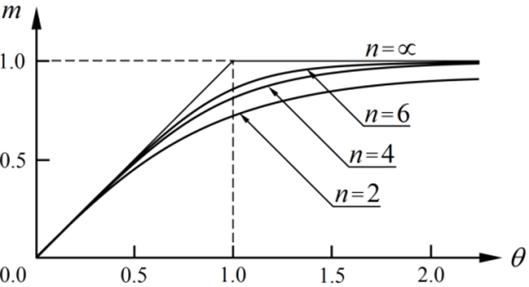Figure 2. Nondimensional representation of three-parameter power model.

4. Determination of Ultimate Connection Moment

4.1. Modeling Technique

The moment-rotation model proposed by Kishi and Chen  is used with refinement based on an improved modeling technique of connection components accounting to revised locations of plastic hinges, bolt stiffness and prying action in failure mechanisms to calculate required ultimate connection moment for proposed connection model which, here in after, will be treated as ‘modified three-parameter power model’ or in short ‘modified power model’.

4.1.1. Assumptions

The following assumptions are employed in determination of connection’s ultimate moment capacity for modified power model; some of which were applied in Kishi-Chen’s power model .

(a)  Center of rotation of a deformed connection is located at the intersecting point of the horizontal middle plane and the vertical cross-section at the toe of the fillet of angle leg adjacent to the compression beam flange (point C in Figure 3);

(b)  Deformations of connection elements are small;

(c)  Materials of connecting elements are elasto-plastic;

(d)  One plastic hinge is assumed to form at the angle’s leg adjacent to compression beam flange at the center of rotation (H4 in Figure 3) with other plastic hinges in the tension angle and tension fasteners at the ultimate level of loading.

(e)  Connecting bolts are at least so strong that the tension bolts could fail simultaneously with tension angle at the stage of connection failure.

4.1.2. Failure Mechanisms

Following the T-stub model , two types of mechanisms of connection failure are considered to evaluate the ultimate moment capacity. These mechanisms have been incorporated from deformed shapes of experimented connections as reported by Azizinaminiet et al.  and Harper , and the places of some plastic hinges are revised from those assumed in the failure mechanism of Kishi-Chen’s power model . These mechanisms are verified by the deformed shapes and plastic yielding areas of connections obtained from nonlinear FE analyses [3,5] and experiments [5-8,15]. The assumed mechanisms of the tension angle and bolt at the time of failure of the connections are

Mechanism Type I

Two plastic hinges are assumed to develop in the tension angle, and tension bolts are considered as stiffer members than tension angle (similar to Kishi-Chen’s model). In this model, the location of formation of the upper plastic hinge is revised from Kishi-Chen’s assumption based on the results obtained from 3D FE analyses [4,5] and experimental studies [5-8,15]. And it is assumed for this type of mechanism that the prying force contributes to form the upper plastic hinge of the tension angle.

Mechanism Type II

One plastic hinge is assumed to form in the tension angle and another in the bolt shank due to the combined action of bending and tensile forces. This type is considered to cover the case in which yielding occurs simultaneously in the tension angle and the tension bolt and prying action contributes to the yielding.

These two simple failure mechanisms in the case of the fastening bolts being arranged in one line are shown in Figures 3 and 4.

In these mechanisms, it is assumed that the prying force develops in between the centerline of bolt hole and the top edge of the tension angle; and the prying force and beam flange force (hereinafter, indicated as shear force) are determined considering the location of the prying force and the plastic moment capacity at the plastic hinges of the tension angle. The smallest shear force among those estimated from these two mechanisms is taken as the shear resistance of a given connection and is used to evaluate the ultimate moment capacity of that connection.

4.1.3. Moment-Shear Interaction

Shear resisting forces acting on the plastic hinges of the tension angle corresponding to the Type I and Type II mechanisms can be evaluated by applying Drucker’s moment-shear interaction . According to Drucker’s yield criterion , yielding of the tension angle’s vertical leg occurs under the combined action of bending moment Mt and shear force Vt when the following condition is satisfied: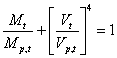(5)

where Mp,t is the pure plastic moment of the tension angle’s vertical leg. According to the maximum shear stress criterion, also known as Tresca’s yield criterion, the pure plastic moment of the tension angle is given by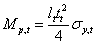(6)

where lt is the width of the tension angle across the column; sy,t is the yield stress of the tension angle, and Vp,t is the pure plastic shear of the tension angle, which can be obtained by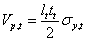(7)

5. Formulation of Resisting Forces and Ultimate Moment Capacity of Connection

5.1. Mechanism Type I

Mechanism Type I is characterized by the formation of three plastic hinges as shown in Figures 3 and 4a.

Applying the work equation and considering moment-shear interaction in the Type I failure mechanism, the shear force can be found by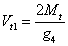(8)

where g4 is the vertical distance between the two plastic hinges, which is revised from Kishi-Chen power model  involved in the failure mechanism of the tension angle (Figure 4a), which can be found by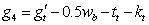(9)

in which gt' is the gage distance from the heel to the centerline of the fastener’s hole in the tension angle’s leg adjacent to the column face; wb is the width of the bolt head across the two opposite flat sides, and kt is the distance from the tension angle’s heel to the toe of the fillet.

Combining Eqs. 6 and 7, the relation between Mp,t and Vp,t can be obtained as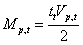(10)

Substituting this relation and Eq. 8 into Eq. 5, a biquadratic equation regarding (Vt1/Vp,t) can be obtained as: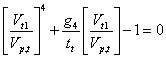(11)

The value of Vt1 can be easily determined by simple iteration of the Eq. 11. A similar equation with the exception of the definition of g4 is derived by Kishi and Chen .

From the equilibrium condition of the tension angle for the Type I mechanism, the tension resistance of the fasteners can be obtained by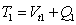(12)

in which Q1 is the prying force for the Type I mechanism. From the condition of plastification of the tension angle’s vertical leg at the plastic hinge H1 (Figure 4a), the prying force is given by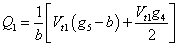(13)

where g5 is the distance from the plastic hinge H1 to the location of the prying force (Figure 4a) and is defined by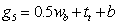(14)

and b is the distance from the centerline of the fastener’s hole to the location of the prying force at the ultimate level of loading. Distance b was investigated previously by conducting FE analyses of top- and seat-angle connections and approximated with some conservative provision by Eq. 1.

The ultimate moment capacity Mu of the top- and seat- angle connection, obtained by taking moment about the center of rotation (Figures 3 and 4a), can be estimated by the following equation: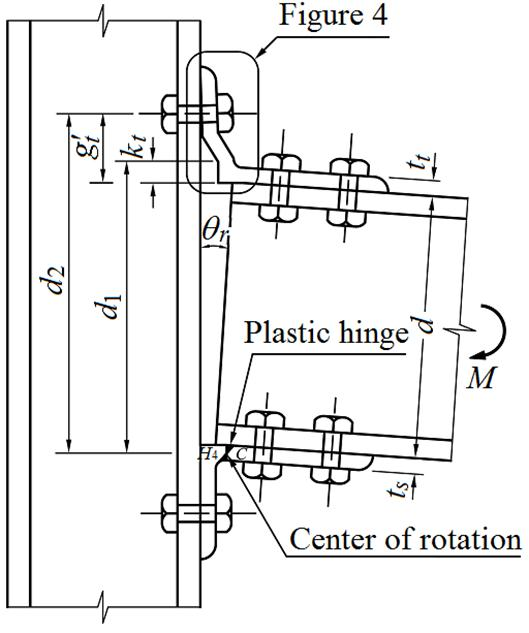Figure 3. Deformed configuration of top- and seat-angle connection.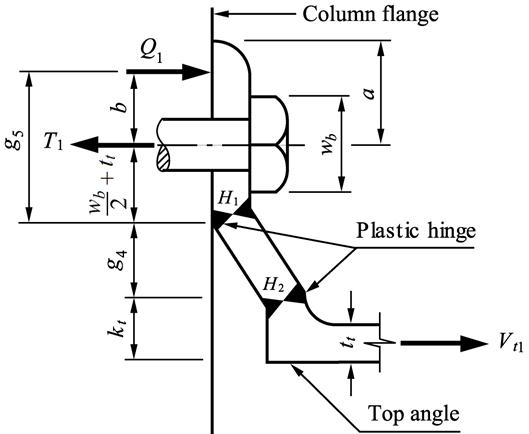a) Type I mechanism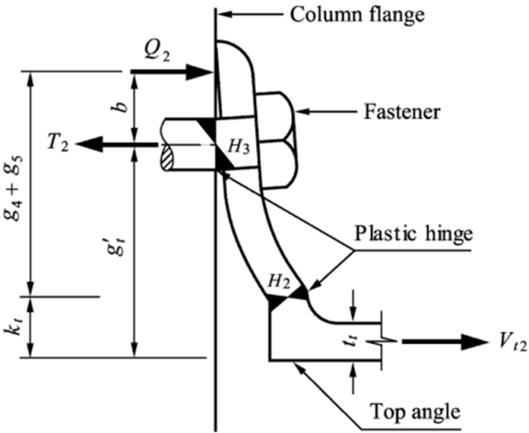b) Type II mechanism

Figure 4. Failure mechanisms of tension angle.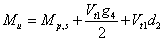(15)

in which Mp,s is the pure plastic moment of the seat angle’s leg adjacent to the compression beam flange, which ignores the interaction with the axial force and can be determined by Eq. 6 employing ts and sy,s of the seat angle in lieu of tt and sy,t. The distance d2 is from the center of rotation to the plastic hinge H2 (Figures 3 and 4a), which can be found by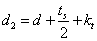(16)

5.2. MechanismType II

Mechanism Type II is shown in Figures 3 and 4b. Considering the moment-shear interaction effect for this mechanism, the plastic yielding of the tension angle’s vertical leg at the plastic hinge H2 (Figure 4b) provides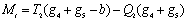(17)

where distances g4, g5 and b can be determined from Eqs. 9, 14 and 1, respectively; T2 and Q2 are the tension resistance of the fastener and the prying force, respectively for Type II mechanism.

Combining Eqs. 10 and 17 with Drucker’s moment-shear interaction Eq. 5, the condition of plastification of the tension angle (Figure 4b) can be written in the following form: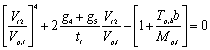(18)

where Tp,b is the axial tensile resisting capacity of the bolts’ shank, but not of the threaded area of the shank because yielding of tension fasteners occurs at the shank area near the fastener’s head due to bending of the shank (explained previously), which is given by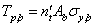(19)

where nt' is the number of fasteners in the tension angle’s leg adjacent to the column; Ab is the cross-sectional area of the fasteners’ shank, and σy,b is the yield stress of fastener’s material.

Substituting the nondimensional values μ = (g4 + g5)/tt and η = 1 +Tp,bb/Mp,t into Eq. 18, the following equation for shear resistance of the tension angle can be obtained: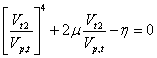(20)

where Vt2 can be determined from Eq. 20 by a simple iteration procedure.

The tension resistance of the fastener is given by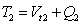(21)

By applying Eq. 21 and substituting Mp,t in lieu of Mt in Eq. 17, the prying force developed in the tension fasteners can be expressed with some conservatism as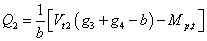(22)

Taking the moment of all forces developed in the plastic hinges for mechanism Type II about the center of rotation, the ultimate moment capacity of the connection can be obtained by

Mu= Mp,s+ Mp,b + Vt2d2                (23)

where Mp,b is the pure plastic moment of the fasteners. Ignoring the interaction with the axial force, it is given by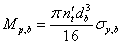(24)

where db is the fastener diameter.

6. Assessment of Proposed Prediction Model

The three-parameter power model  drew attention of many researchers for M-qr modeling of top- and seat-angle connections. The model has been refined here by proposing an improved method of determining ultimate moment capacity Mu of top- and seat-angle connections. The initial connection stiffness Rki of the connection is determined by using the same method as proposed by Kishi and Chen  in their three-parameter power model, and the shape parameter n is determined by employing a least-mean square fit technique of the data-base program SCDB . Three experiments of top- and seat-angle connection stored in updated databank  are used to conduct a comparison among results of experiments, FE analyses, power model of Kishi and Chen , and modified power model. The procedure of assessment of the M-qr curves is conducted with the help of computer program SCDB . The effective mechanical properties of the material of the connection components used for predicting M-qr curves by the proposed prediction model and the three-parameter power model are shown in Table 1. A summary of the assessment of theproposed prediction model is shown in Table 2 and comparisons of the connections are shown in Figures 5 and 6.

It is observed from Table 2 that the proposed modified power model predicts somewhat larger ultimate moment capacity than that of the power model because of revision of the vertical gage distance between the two plastic hinges developed in tension angle for test connection models A1 (Figure 5a) and FE models FE5, FE6 and FE9 (Figures 6 e, f and i). It is also able to decrease the ultimate moment capacity of connections FE1, FE4, FE7 and FE11 (Figures 6 a, d, g and k) significantly because of consideration of bolt stiffness and prying action in the proposed prediction model, and shows close fit with the experimental and/or FE analysis results.

Table 1. Mechanical properties of connection elements used for estimating Mu in proposed modified power model and Kishi-Chen power model.

 Test or FE model Top and seat angles Bolt Yield stress, σy [MPa] Yield stress, σyb [Mpa] Connections tested by Azizinamini et al.  A1, A2 301 635 Connection tested by Harper  Test 3 297 635 Connection tested by Davision et al.  JT/08 259 635 FE analysis of top- and seat-angle connections  FE1~FE6, FE9~FE13 365 635 FE7 250 FE8 365 830

Table 2. Assessment of the proposed prediction model.

 Model or Test ID Test Power model Proposed prediction model FE analysis Mu [kNm] Mu [kNm] Vp [kN] Mu [kNm] Vt [kN] Q [kN] T [kN] Mu [kNm] Vt [kN] Q [kN] T [kN] Connections tested by Azizinamini et al.  A1 70.3 68.7 171.2 71.1 177.5 287.1 464.6 75.2 190.0 238.7 432.8 A2 95.8 109.0 267.8 101.2 245.6 233.3 478.8 109.6 269.3 212.2 481.5 Connection tested by Harper  Test 3 45.1 50.0 206.0 51.0 210.6 270.0 480.6 54.8 226 159.8 406.6 Connection tested by Davision et al.  JT/08 23.6 35.1 125.1 35.4 125.1 94.9 231.4 - - - - FE connection models  FE1 - 236.3 563.3 153.0 358.5 122.0 480.5 154.9 363.7 155.3 519.0 FE2 - 132.7 338.6 97.2 240.2 235.6 475.8 98.0 254.7 187.3 442.0 FE3 - 23.3 51.7 23.6 52.4 166.8 219.2 45.7 109.4 253.6 385.8 FE4 - 113.9 276.9 83.1 200.7 157.6 358.2 66.3 167.2 159.5 326.7 FE5 - 83.3 207.7 78.7 192.3 292.9 485.2 75.2 190.2 238.5 431.0 FE6 - 83.3 207.7 78.7 192.3 292.9 485.2 75.9 191.8 237.0 434.3 FE7 - 160.5 382.7 135.8 320.9 147.3 468.2 128.9 306.7 181.4 488.1 FE8 - 132.2 324.8 129.9 315.7 305.9 621.6 115.4 285.2 279.1 564.3 FE9 - 51.0 207.7 48.7 192.3 292.9 485.2 44.5 180.8 241.6 430.6 FE10 - 81.7 324.8 65.8 255.3 228.1 483.4 66.1 260.8 212.3 473.1 FE11 - 148.6 563.3 97.2 358.5 122.0 480.5 95.6 350 162.4 512.4 FE12 - 79.9 338.6 59.8 240.2 235.6 475.8 56.7 240.3 208.9 449.2 FE13 - 15.3 51.7 15.4 52.4 166.8 219.2 26.6 96.8 236.4 358.7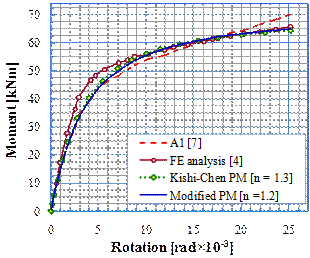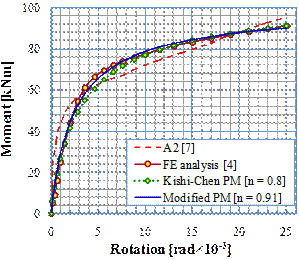a) Test A1                                                                      b) Test A2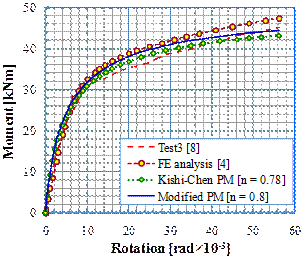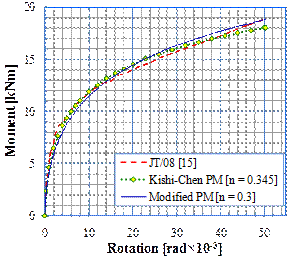c) Test 3                                                                      d) JT/08

Figure 5. Performances of modified power model and Kishi-Chen three-parameter power model.

FE analyses showed that large deformation of the tension angle is produced for the connections with long gage (gt'), such as for FE3 and FE13 connections and the small deformation criteria considered for proposed formulation do not allow predicting accurately the ultimate moment capacity of the connections (Figures 6c and m). The studies of Yang and Tan found the similar observation of that the angles with large gage, gt' deform significantly in tension and develop stiffer strain-hardening characteristic in moment-rotation behavior after large rotations . It is obvious in Figures 5 and 6 that the M-qr curves predicted by the proposed modified power model and Kishi-Chen power model have good agreement with FE analysis and experimental data in the elastic region. But the results of the both models differ more from the experimental and FE analysis M-qr curves in the plastic region as the moment increases to the ultimate level. This mainly occurs due to that the three-parameter power model (Eq. 2) avoided considering strain-hardening stiffness in the connection modeling. It is also shown in Table 2 that the proposed prediction model estimates closer values of ultimate moment capacity of top- and seat-angle connections to those measured in experiments and calculated by FE analysis. The proposed prediction model is also able to estimate shear-resisting force Vt of the tension angle and tensile resisting forces T of bolts, which are also compared with FE analysis results (Table 2). The assessment shows that the resisting forces of the top angle and tension fasteners estimated by the proposed modified power model are in satisfactory tolerances comparing with those of FE analyses.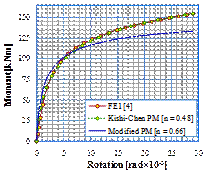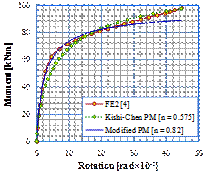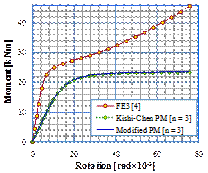a) FE1                                    b) FE2                                     c) FE3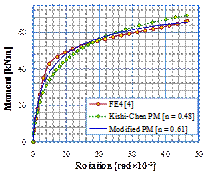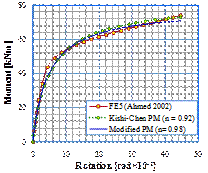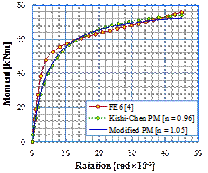d) FE4                                    e) FE5                                     f) FE6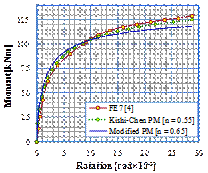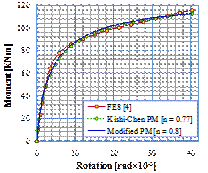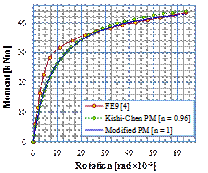g) FE7                                    h) FE8                                     i) FE9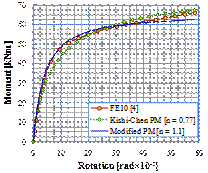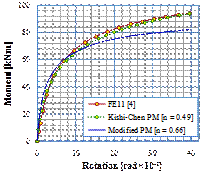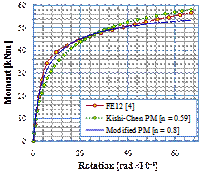j) FE10                                    k) FE11                                    l) FE12

Figure 6. Verification of modified power model and Kishi-Chen three-parameter power model.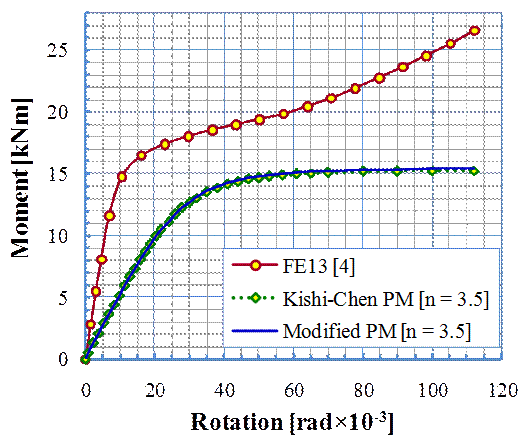m) FE13

Figure 6. Continue.

7. Prediction Equation for Shape Parameter

To predict moment-rotation curves of top- and seat-angle connections efficiently, it is necessary to readjust the Kishi- Chen formulation  of shape parameter n for the proposed mechanisms. The equations of shape parameter n of top- andseat-angle connection are evaluated by undergoing the following procedure:

(a)  The value of n is determined for each test numerically by applying least-mean square fit technique using Eq. 2 .

(b)  Numerical values of n so obtained are then plotted against –log10qo (Figure 7). The expression of estimating shape parameter n is assumed to be a nonlinear function of log10qo obtained by a second-order nonlinear regression analysis from the n log10qo graph to have the best fit with the proposed modified power model.

(c)  The above procedure results in two empirical equations for estimating the design values of shape parameter n of top- and seat- angle connections of mechanisms Type I and Type II. They are expressed for

Mechanism Type I

n = − 2.25(log10qo)2 – 6(log10qo) − 0.47      (25)

Mechanism Type II

n = − 0.322(log10qo) + 1.67             (26)

Figures 7a and b illustrate the distribution of n values calculated M-qr curve by Eq. 25 and 26 comparing with those of experiments and FE analyses.

8. Conclusions

Investigating the places of plastic hinge formation by conducting 3D finite element analyses and considering the failure modes identified by available experiments, two simple failure mechanisms were assumed for top- and seat-angle connections. Using the simple mechanisms of connection failure, a method of estimating the ultimate moment capacity has been proposed for top - and seat - angle connections considering prying force, bolt stiffness and tension angle’s bending deformation. The performance of the proposed modified power model along with Kishi-Chen power model was verified by comparing the ultimate moment capacity and M-qr curves predicted by the proposed model and Kishi-Chen power model with experimental and FE results. The assessment of the proposed model reveals that both proposed and Kishi-Chen power models have the same level of accuracy in predicting M-qr relations, but the proposed modified power model shows better performance in estimating the ultimate moment capacities of the connections than that of Kishi-Chen power model. Therefore, the performance of Kishi-Chen power model has been improved by the refinement brought in the proposed modified power model.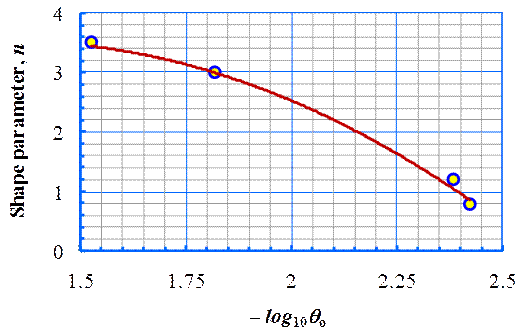a) Mechanism Type I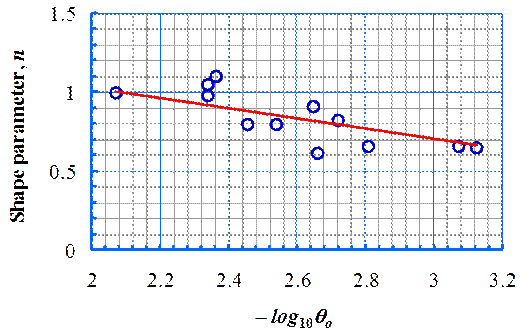b) Mechanism Type IIProposed equation,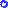Calculated for experiments and FE analyses

Figure 7. Comparison of equations of shape parameter with the produced values of experiments and FE analyses.

This study also revealed that the proposed model could perform better if strain-hardening stiffness and large deformation for connections (such as FE3 and FE13) with long gage, gt' are considered in moment-rotation modeling of top- and seat-angle connections.

References

1. N. Kishi and W. F. Chen, "Moment- rotation relations of semi-rigid connections with angles." Journal of Structural Engineering, ASCE, vol. 116 (7), 1990, pp. 18131834.
2. CEN, Eurocode 3, ENV 1993-1-1, Design of Steel Structures. Annex J, Part 1. 1: Joint in Building Frames. European Committee of Standardization, Brussels, 1997.
3. A. Ahmed, N. Kishi, K. G. Matsuoka and M. Komuro, "Nonlinear analysis on prying of top- and seat-angle connections." Journal of Applied Mechanics, JSCE, vol. 4, 2001, pp. 227−236.
4. A. Ahmed, "Finite element analysis and modeling of M-qrrelation for connections with angles", Ph. D. thesis, Department of Civil Engineering and Architecture, Muroran Institute of Technology, Japan, March, 2002.
5. M. Komuro, N. Kishi and W. F. Chen, "Elasto-plastic FE analysis on moment-rotation relations of top-and seat-angle connections", 5th International Workshop on Connections in Steel Structures: Behaviour, Strength and Design, Faculty of Civil Engineering and Geosciences of the Delft University of Technology, Amsterdam, 2004, pp. 111-120.
6. A. Pirmoz, A. S. Khoei, E. M. Rezapour and A. S. Daryan, "Moment-rotation behaviour of bolted top-seat angle connections", Journal of Constructional Steel Research, vol. 65, 2009, pp. 973-984.
7. A. Azizinamini, J. H. Bradburnand J. B. Radziminski, "Static and cyclic behavior of semi-rigid steel beam-column connections", Structural research studies, Department of Civil Engineering, University of South Carolina, Columbia, S. C., March, 1985.
8. W. L. Harper, "Dynamic response of steel frames with semi- rigid connections", Structural research studies, Department of Civil Engineering, University of South Carolina, Columbia, S. C., May, 1990.
9. A. Abolmaali, A. Kukreti, A. Motahari and M. Ghassemieh, "Energy dissipation characteristics of semi-rigid connections", Journal of Constructional Steel Research, vol. 65, no. 5, 2009, pp. 1187-1197.
10. T. Beland et al., "Experimental behavior of bolted angles and beam-column connections", Proceedings of the Structural Congress 2014, ASCE, Boston, MA, April 3-5, 2014, pp. 2405-2416.
11. K. Weynand, J. P. Jaspart and M. Steenhuis, "The stiffness model of revised Annex J of Eurocode 3", In: Bjorhovde R., Colson A., Zandonini R., editors, "Connections in steel structures III", Proceedings of the 3rd international workshop on connections in steel structures, 1995.
12. C. Faella, V. Piluso and G. Rizzano, "Prediction of the flexural resistance of bolted connections with angles", IABSE Colloquium on Semirigid Structural Connections, Istanbul, September, 1996, pp. 25−27.
13. A. M. Citipitioglu, R. M. Haj-Ali and D. W. White, "Refined 3D finite element modeling of partially restrained connections including slip", Journal of Constructional Steel Research, vol. 58 (5-8), 2002, pp. 995-1013.
14. N. Kishi and W. F. Chen, "Data base of steel beam-to-column connections", Structural Engineering, Report No. CE-STR-86-26, School of Civil Engineering, Purdue University, West Lafayette, IN, 1986.
15. J. B. Davison, P. A. Kirby and D. A. Nethercot, "Rotational stiffness characteristics of steel beam-to-column connections", Journal of Constructional Steel Research, vol. 8 (C), 1987, pp. 17-54.
16. A. Ahmed and R. Hasan, "Effect and Evaluation of Prying Action for Top- and Seat-Angle Connections", International Journal of Advanced Structural Engineering, vol. 7, no. 2, 2015, pp. 159-169.
17. J. C. Rathbun, "Elastic properties of riveted connections", Trans. ASCE, no. 1933, 101, 1936, pp. 524−563.
18. A. R. Monforton and T. S. Wu, "Matrix analysis of semi-rigidly connected frames", Journal of the Structural Division, ASCE, vol. 87, no. 6, 1963, pp. 13−42.
19. E. Lightfoot and A. P. LeMessurier, "Elastic analysis of frameworks with elastic connections", Journal of the Structural Division, ASCE, vol. 100, no. 6, 1974, pp. 1297−1309.
20. T. S. Tarpy and J. W. Cardinal, "Behavior of semi-rigid beam-to-column end plate connections", Joints in Structural Steelwork, J. H. Howlett et al., eds, Pentech Press, London, 1981, pp. 2.3−2.25.
21. E. M. Lui and W. F. Chen, "Strength of H-columns with small end restraints", Journal of the Institution of Structural Engineers, London, vol. 61B(1), 1983, pp. 17−26.
22. S. W. Jones, P. A. Kirby and D. A. Nethercot, "Effect of semi-rigid connections on steel column strength", Journal of Construction Steel Research, vol. 1, no. 1, 1980, pp. 38−46.
23. S. W. Jones, P. A. Kirby and D. A. Nethercot, "Modeling of semi-rigid connection behavior and its influence on steel column behaviour", Joints in Structural Steelwork, J. H. Howlett, W. M. Jenkins, and R. Stainsby, eds, Pentech Press, London, 1981, pp. 5.73−5.78.
24. M. J. Frye and G. A. Morris, "Analysis of flexibly connected steel frames", Canadian J. of Civil Engineers, vol. 2 (3), 1975, pp. 280291.
25. E. M. Lui, and W. F. Chen, "Analysis and behavior of flexibly jointed frames", Engineering Structures Journal, Buttersworth, U. K., vol. 8, 1986, pp. 107−118.
26. R. M. Richard and B. J. Abbott, "Versatile elastic-plastic stress-strain formula", Journal of Engineering Mechanical Division, ASCE, vol. 101 (4), 1975, pp.511−515.
27. A. Colson and J. M. Louveau, "Connections Incidence on the Inelastic Behavior of Steel Structures", Euromech Colloguium 174, 1983.
28. D. C. Drucker, "The effect of shear on the plastic bending of beams", Journal of Applied Mechanics, vol. 23 (4), 1956, pp. 509514.
29. W. F. Chen and N. Kishi, "Semi-rigid steel beam-to-column connections: Data Base and Modeling", Journal of Structural Engineering, ASCE, vol. 115 (1), 1989, pp. 105119.
30. B. Yang, and K. Tan, "Behavior of Composite Beam-Column Joints in a Middle-Column-Removal Scenario: Experimental Tests", Journal of Structural Engineering, ASCE, vol. 140, No. 2, February, 2014.

 Contents 1. 2. 3. 4. 4.1. 5. 5.1. 5.2. 6. 7. 8.
Article ToolsAbstractPDF(615K)